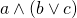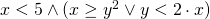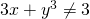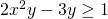## Practical Course: SMT solving for real arithmetic with ICP

Satisfiability Checking is the task of checking the existence of a satisfying solution for a logical formula. For propositional logic formulas, being Boolean combinations of propositions such as, SAT solvers can be used to determine whether there is an assignment mapping to each proposition a value either true or false, such that substituting these values into the formula, the formula evaluates to true.

For the satisfiability check of quantifier-free first-order logic formulas over different theories, SAT solvers can be extended with theory solver modules, resulting in SAT-modulo-theories (SMT) solvers. Thereby we move from checking satisfiability for propositional logic formulas, such as, towards checking satisfiability for formulas such as. Such logics can be syntactically more rich as well as semantically more expressive than propositional logic, and allow the natural formalisation of a wide range of practical problems, e.g., in scheduling, planning, software verification, or controller synthesis.

This practical course aims at the design, implementation, and optimization of state-of-the-art decision procedures for SMT solving for the theory of real arithmetic. This theory allows for arbitrary equalities and inequalities over polynomials containing real variables, for exampleor.
One particular approach for this theory that is not complete but performs very well in many cases is interval constraint propagation (ICP). It over-approximates the solution space by a box and narrows this box without losing solutions until either this box becomes empty (and thus no solution exists) or the size of the box is below some threshold. Then, we can try to guess a solution from the very small box, or even use another decision procedure that can make use of the reduced search space.

We will start with the implementation of the basic ICP algorithm and continue with optimizations in the second half of the practical course.

The implementation will take place within the SMT-RAT project and can benefit from its manifold features. The resulting SMT solver will be tested on the official SMT-LIB benchmarks and be compared to state-of-the-art SMT solvers. Furthermore, we will form at least two teams and compare their solvers within a competition.